# Chapter 4 – Geometry -Additional Questions.

10 Dec 2020 3:14 pm

Tamilnadu, Samacheer, Kalvi, 10th, sslc, Maths, Solutions, Chapter 4, Geometry, Additional, Questions,

Question 1.
If a straight line intersects the sides AB and AC of a ∆ABC at D and E respectively and is parallel to BC, then AEAC = …………
(3) DEBC

Hint:
By BPT theorem,

Question 2.
In ∆ABC, DE is || to BC, meeting AB and AC at D and E. If AD = 3 cm, DB = 2 cm and AE = 2.7 cm, then AC is equal to ……..
(1) 6.5 cm
(2) 4.5 cm
(3) 3.5 cm
(4) 3.5 cm
(2) 4.5 cm
Hint:
By BPT theorem,

32 = 2.7EC
∴ EC = 2.7×23 = 1.8 CM
AC = AE + EC
= 2.7 + 1.8 = 4.5 cm

Question 3.
In ∆PQR, RS is the bisector of ∠R. If PQ = 6 cm, QR = 8 cm, RP = 4 cm then PS is equal to …………..
(1) 2 cm
(2) 4 cm
(3) 3 cm
(4) 6 cm
(2) 2 cm
Hint:

By ABT theorem,
PSSQ = PRQR ⇒ x6−x = 48
24 – 4x = 8x ⇒ 24 = 12x
x = 2
PS = 2 cm

Question 4.
In figure, if ABAC = BDDC, ∠B = 40° and ∠C = 60°, then ∠BAD = ……………
(1) 30°
(2) 50°
(3) 80°
(4) 40°
(4) 40°
Hint:
ABAC = BDDC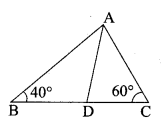AD is the internal bisector of ∠BAC
∠A + ∠B + ∠C = 180°
∠A + 40° + 60° = 180° ⇒ ∠A = 80°
∴ ∠BAC = 802 = 40°

Question 5.
In the figure, the value x is equal to …………
(1) 4.2
(2) 3.2
(3) 0.8
(4) 0.4

(2) 3.2
Hint:
By Thales theorem
(DE || BC)
x8 = 410 ⇒ x = 8×410 = 3.2

www.asterclasses.com

Question 6.
In triangles ABC and DEF, ∠B = ∠E, ∠C = ∠F, then ………….
(1) ABDE = CAEF
(2) BCEF = ABFD
(3) ABDE = BCEF
(4) CAFD = ABEF
(3) ABDE = BCEF
Hint:

Question 7.
From the given figure, identify the wrong statement.

(2) ∆ABD ~ ∆ABC
(3) ∆BDC ~ ∆ABC
(2) ∆ ABD ~ ∆ ABC

www.asterclasses.com

Question 8.
If a vertical stick 12 m long casts a shadow 8 m long on the ground and at the same time a tower casts a shadow 40 m long on the ground, then the height of the tower is …………..
(1) 40 m
(2) 50 m
(3) 75 m
(4) 60 m
(4) 60 m
Hint:

ABDE = BCEF
12h = 840; h = 40×128 = 60

Question 9.
The sides of two similar triangles are in the ratio 2:3, then their areas are in the ratio ………….
(1) 9 : 4
(2) 4 : 9
(3) 2 : 3
(4) 3 : 2
(2) 4 : 9
Hint:
Ratio of the Area of two similar triangle
= 22 : 32 = 4 : 9
[squares of their corresponding sides]

Question 10.
Triangles ABC and DEF are similar. If their areas are 100 cm2 and 49 cm2respectively and BC is 8.2 cm then EF = …………….
(1) 5.47 cm
(2) 5.74 cm
(3) 6.47 cm
(4) 6.74 cm
(2) 5.74 cm
Hint:

Question 11.
The perimeters of two similar triangles are 24 cm and 18 cm respectively.
If one side of the first triangle is 8 cm, then the corresponding side of the other triangle is ………
(1) 4 cm
(2) 3 cm
(3) 9 cm
(4) 6 cm
(4) 6 cm
Hint:

Question 12.
A point P is 26 cm away from the centre O of a circle and PT is the tangent drawn from P to the circle 10 cm, then OT is equal to …………
(1) 36 cm
(2) 20 cm
(3) 18 cm
(4) 24 cm
(4) 24 cm
Hint:

OT2 = OP2 – PT2
= 262 – 102
= (26 + 10) (26 – 10)
OT2 = 36 × 16
OT = 6 × 4 = 24 cm

Question 13.
In the figure, if ∠PAB = 120° then ∠BPT = ……….

(1) 120°
(2) 30°
(3) 40°
(4) 60°
(4) 60°
Hint:
∠BCP + ∠PAB = 180°
(sum of the opposite angles of a cyclic quadrilateral)
∠BCP = 180° – 120° = 60°
∠BPT = 60°
(By tangent chord theorem)

Question 14.
If the tangents PA and PB from an external point P to circle with centre O are inclined to each other at an angle of 40°, then ∠POA = ………………
(1) 70°
(2) 80°
(3) 50°
(4) 60°
(1) 70°
Hint:
∠OPA = 402 = 20°
In ∆OAP.
∠POA + ∠OAP + ∠APO = 180°
(sum of the angles of a triangle)

∠POA + 90° + 20° = 180°
∠POA = 180° – 110° = 70°

Question 15.
In the figure, PA and PB are tangents to the circle drawn from an external point P. Also CD is a tangent to the circle at Q. If PA = 8 cm and CQ = 3 cm, then PC is equal to ……….

(1) 11 cm
(2) 5 cm
(3) 24 cm
(4) 38 cm
(2) 5 cm
Hint:
PA = PB (tangent of a circle)
PB = 8 cm
PC + BC = 8
PA + QC = (BC = QC tangent)
PC + 3 = 8
∴ PC = 8 cm – 3 cm = 5 cm

Question 16.
∆ABC is a right angled triangle where ∠B = 90° and BD ⊥ AC. If BD = 8 cm, AD = 4 cm, then CD is …………
(1) 24 cm
(2) 16 cm
(3) 32 cm
(4) 8 cm
(2) 16 cm
Hint:
∆DCB ~ ∆DBA
DCDB = DBDA

DB2 = DC × DA
82 = DC × 4
DC = 644 = 16 cm

Question 17.
The areas of two similar triangles are 16 cm2 and 36 cm2 respectively. If the altitude of the first triangle is 3 cm, then the corresponding altitude of the other triangle is
(1) 6.5 cm
(2) 6 cm
(3) 4 cm
(4) 4.5 cm
(4) 4.5 cm
Hint:

Question 18.
The perimeter of two similar triangles ∆ABC and ∆DEF are 36 cm and 24 cm respectively. If DE =10 cm, then AB is …………
(1) 12 cm
(2) 20 cm
(3) 15 cm
(4) 18 cm
(3) 15 cm
Hint:
Perimeter of

Question 19.
In the given diagram θ is ………….
(1) 15°
(2) 30°
(3) 45°
(4) 60°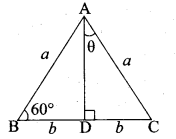(2) 30°
Hint:
∠BAD = 180° – 150° = 30°
= 180° – 150° = 30°
∠DAC = θ = 30°

Question 20.
If AD is the bisector of ∠A then AC is …………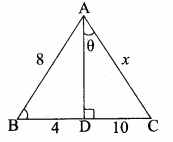(1) 12
(2) 16
(3) 18
(4) 20
(4) 20
Hint. In ∆ABC, AD is the internal bisector of ∠A
BDDC = ABAC
410 = 8x
4x = 10 × 8
x = 10×84 = 20 cm

Question 21.
In ∆ABC and ∆DEF, ∠A = ∠E and ∠B = ∠F. Then AB : AC is ………….
(1) DE : DF
(2) DE : EF
(3) EF : ED
(4) DF : EF
(3) EF : ED
Hint:

ABEF = BCFD = ACED

Question 22.
Two circles of radius 8.2 cm and 3.6 cm touch each other externally, the distance between their centres is ………….
(1) 1.8 cm
(2) 4.1 cm
(3) 4.6 cm
(4) 11.8 cm
(4) 11.8 cm
Hint:
Distance between the two centres

= r1 + r2
= 8.2 + 3.6
= 11.8 cm

Question 23.
In the given diagram PA and PB are tangents drawn from P to a circle with centre O. ∠OPA = 35° then a and b is …………

(1) a = 30°, b = 60°
(2) a = 35°, b = 55°
(3) a = 40°, b = 50°
(4) a = 45°, b = 45°
(2) a = 35°, b = 55°
Hint:
∠OAP = 90° (tangent of the circle)
∠AOP + ∠OPA + ∠PAO = 180°
b + 35° + 90° = 180°
b = 180° – 125°
= 55°
OP is the angle bisector of ∠P
∴ a = 35°

### II. Answer the following questions

Question 1.
The image of a man of height 1.8 m, is of length 1.5 cm on the film of a camera. If the film is 3 cm from the lens of the camera, how far is the man from the camera?
Solution:
Let AB be the height of the man, CD be the height of the image of the man of height 1.8 m (180 cm). LM be the distance between man and lens. LN be the distance between Lens and the film.

Given, AB = 1.8 m (180 cm)
CD = 1.5 cm and LN = 3 cm
Consider ∆ LAB and ∆ LCD
∠ALB = ∠DLC (vertically opposite angles)
∠LAB = ∠LDC (alternate angles) (AB || CD)
∴ ∆ LAB ~ ∆ LDC (AA similarity)
∴ ABCD = LMLN ⇒ 1801.5 = x3 ⇒ 1.5x = 180 × 3
x = 180×31.5 = 180×3×1015
x = 360 cm (or) 3.6 m
∴ The distance between the man and the camera = 3.6 cm

Question 2.
A girl of height 120 cm is walking away from the base of a lamp-post at a speed of 0. 6 m/sec. If the lamp is 3.6 m above the ground level, then find the length of her shadow after 4 seconds.
Solution:
Let AB be the height of the lamp-post above the ground level.
AB = 3.6 m = 360 cm
Let CD be the height of the girl.
CD = 1.2 m = 120 cm
The distance travelled by the girl in 4 seconds (AC)
= 4 × 0.6 = 2.4m = 240 cm
Consider ∆ECD and ∆EAB
Given (CD || AB)
∠EAB = ∠ECD = 90°
∠E is common

∴ ∆ EAB = ∆ ECD = 90°
ABCD = AECE
= x+240x ⇒ 3 = x+240x
3x = x + 240
2x = 240 ⇒ x = 2402 = 120 cm
∴ Lenght of girls shadow after 4 seconds = 120 cm

Question 3.
In ∆ ABC, AB = AC and BC = 6 cm. D is a point on the side AC such that AD = 5 cm and CD = 4 cm. Show that ∆BCD ~ ∆ACB and hence find BD.
Solution:
Given, In ∆ ABC, AB = AC = 9 cm and BC = 6 cm, AD = 5 cm and CD = 4 cm
BCAC = 69 = 23 …..(1)
CDCB = 46 = 23 ……..(2)

From (1) and (2) we get,
BCAC = CDCB
In ∆ BCD and ∆ ACB
∠C = ∠C (common angle)
BCAC = CDCB
∴ ∆ BCD ~ ∆ ACB
BDAB = BCAC ⇒ BD9 = 69 ⇒ ∴ BD = 6 cm.

Question 4.
The lengths of three sides of a triangle ABC are 6 cm, 4 cm and 9 cm. ∆PQR ~ ∆ABC. One of the lengths of sides of APQR is 35 cm. What is the greatest perimeter possible for ∆PQR?
Solution:
Given, ∆ PQR ~ ∆ ABC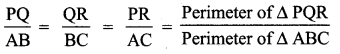Perimeter of ∆ ABC = 6 + 4 + 9 = 19 cm
When the perimeter of ∆ PQR is the greatest only the corresponding side QR must be equal to 35 cm

Question 5.
A man sees the top of a tower in a mirror which is at a distance of 87.6 m from the tower. The mirror is on the ground, facing upward. The man is 0.4 m away from the mirror, and the distance of his eye level from the ground is 1.5 m. How tall is the tower? (The foot of man, the mirror and the foot of the tower lie along a straight line).
Solution:
Let AB and ED be the heights of the man and the tower respectively. Let C be the point of incidence of the tower in the mirror.
In ∆ ABC and ∆ EDC, we have

www.asterclasses.com

Question 6.
In ∆ PQR, given that S is a point on PQ such that ST || QR and PSSQ = 35. If PR = 5.6 cm, then find PT.
Solution:
In ∆ PQR, we have ST || QR and by Thales theorem,
PSSQ = PTTR …….(1)
Let PT = x
Thus, TR = PR – PT = 5.6 – x

From (1), we get PT = TR (PSSQ)
x = (5.6 – x) (35)
5x = 16.8 – 3x
8x = 16.8
x = 16.88 = 2.1
That is PT = 2.1 cm

Question 8.
In the figure AC || BD and CE || DF. if OA = 12 cm, AB = 9cm, OC = 8 cm and EF = 4.5 cm, then find FO.
Solution: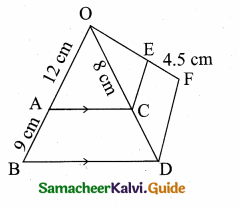In OBD, AC || BD
∴ OAAB = OCCD (By Thales theorem)
129 = 8CD
∴ CD = 9×812 = 6 CM
In ODF, CE || DF
OCCD = OEEF (By Thales theorem)
86 = OE4.5 ⇒ OE = =8×4.56 = 6 cm
FO = FE + EO = 4.5 + 6 = 10.5 cm
∴ The value of FO = 10.5 cm

Question 9.
Check whether AD is the bisector of ∠A of ∆ABC in each of the following.
(i) AB = 4 cm, AC = 6 cm, BD 1.6 cm, and CD = 2.4 cm.
Solution:
BDDC = 1.62.4 = 1624 = 23 …..(1)
ABAC = 46 = 23 ……..(2)
From (1) and (2) we get,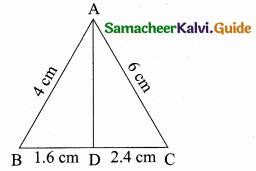BDDC = ABAC
By the converse of angle bisector theorem we have,
∴ AD is the internal bisector of ∠A

(ii) AB = 6 cm, AC = 8 cm, BD = 1.5 cm and CD = 3 cm
Solution:
BDDC = 1.53 = 0.5 …….(1)
ABAC = 68 = 34 …….(2)From (1) and (2) we get,
BDDC ≠ ABAC
Hence AD is not the bisector of ∠A.

Question 10.
In a ∆ABC, AD is the internal bisector of ∠A, meeting BC at D. If AB 5.6 cm, AC = 6 cm and DC = 3 cm, find BC.
Solution:
Given, AB = 5.6 cm, AC = 6 cm, DC = 3 cm
Let BD be x
In ∆ ABC, AD is the internal bisector of ∠A.
By Angle bisector theorem, we have,BDDC = ABAC ⇒ x3 = 5.66
x = 3×5.66 = 2.8 cm
∴ BC = BD + DC = 2.8 + 3 = 5.8 cm
Length of BC = 5.8 cm

Question 11.
In the figure, tangents PA and PB are drawn to a circle with centre O from an external point P. If CD is a tangent to the circle at E and AP = 15 cm, find the perimeter of ∆PCD.
Solution:
We know that the lengths of the two tangents from an exterior point to a circle are equal.
∴ CA = CE, DB = DE and PA = PB
Now, the perimeter of ∆PCD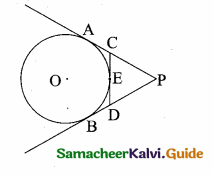= PC + CD + DP
= PC + CE + ED + DP
= PC + CA + DB + DP
= PA + PB = 2 PA (PB = PA)
Thus, the perimeter of APCD = 2 × 15 = 30 cm

Question 12.
ABCD is a quadrilateral such that all of its sides touch a circle. If AB = 6 cm, BC = 6.5 cm and CD = 7 cm, then find the length of AD.
Solution:
Let P, Q, R and S be the points where the circle touches the quadrilateral.
We know that the lengths of the two tangents drawn from an exterior point to a circle are equal. Thus, we have,AP = AS, BP = BQ, CR = CQ and DR = DS
Hence, AP + BP + CR + DR = AS + BQ + CQ + DS
⇒ AB + CD = AD + BC
⇒ AD = AB + CD – BC
= 6 + 7 – 6.5 = 6.5

Question 13.
A man goes 10 m due east and then 24 m due north. Find the distance from the starting point.
Solution:
Let the initial position of the man be “O” and the final position be B.
In the ∆AOB,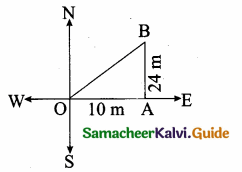OB2 = OA2 + AB2
OB2 = 102 + 242
= 100 + 576 = 676
OB = 676−−−√ = 26 m
The man is at a distance of 26 m from the starting point.

Question 14.
Suppose AB, AC and BC have lengths 13, 16 and 20 respectively. If AFFB = 45 and CEEA= 512 Find BD and DC.
Solution:
Given that AB = 13, AC = 16 and BC = 20. Let BD = JC and DC = y.
Using Ceva’s theorem we haveBDDC × CEEA × AFFB = 1 ………(1)
Substitute the given values
xy × 512 × 45 = 1 ⇒ xy × 13 = 1
xy = 3 ⇒ x = 3y
x – 3y = 0 ……(2)
Given BC = 20
x + y = 20 …..(3)
Subtract (2) and (3)Question 15.
ABC is a right – angled triangle at B. Let D and E be any two points on AB and BC respectively. prove that AE2 + CD2 = AC2 + DE2.
Solution:
In the right ∆ ABE right- angled at B.
AE2 = AB2 + BE2 …..(1)
In the right ∆ DBC,CD2 = BD2 + BC2 ………(2)
Adding (1) and (2) we get
AE2 + CD2 = AB2 + BE2 + BD2 + BC2
= (AB2 + BC2) + (BC2 + BD2)
AE2 + CD2 = AC2 + DE2
Hence it is proved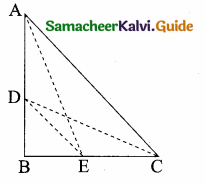[AC2 = AB2 + BC2]
[DE2 = BE2 + BD2]

### III. Answer the following questions

Question 1.
A point O in the interior of a rectangle ABCD is joined to each of the vertices A, B, C and D. Prove that OA2 + OC2 = OB2 + OD2.
Solution:
Given: O is any point inside the rectangle ABCD.
To prove: OA2 + OC2 = OB2 + OD2
Construction: Through “O” draw EF || AB.
Proof: Using Pythagoras theoremIn the right ∆OEA,
∴ OA2= OE2 + AE2 …(1)
(By Pythagoras theorem)
In the right ∆OFC,
OC2 = OF2 + FC2 …(2)
(By Pythagoras theorem)
OB2 = OF2 + FB2 … (3)
(By Pythagoras theorem)
In the right ∆OED,
OD2 = OE2 + ED2 … (4)
(By Pythagoras theorem)
By adding (3) and (4) we get
OB2 + OD2 = OF2 + FB2 + OE2 + ED2
= (OE2 + FB2) + (OF2 + ED2)
= (OE2 + EA2) + (OF2 + FC2)
[FB = EA and ED = FC]
OB2 + OD2 = OA2 + OC2 using (1) and (2)

Question 2.
A lotus is 20 cm above the water surface in a pond and its stem is partly below the water surface. As the wind blew, the stem is pushed aside so that the lotus touched the water 40 cm away from the original position of the stem. How much of the stem was below the water surface originally?
Solution:
Let O be the bottom of the stem immersed in water.
Let B be the lotus, AB be the length of the stem above the water surface
AB = 20 cm
Let OA be the length of the stem below the water surface
Let OA = x cm
Let C be the point where the lotus touches the water surface when the wind blow.
OC = OA + AB
OC = x + 20 cm
In ∆ AOC, OC2 = OA2 + AC2
(x + 20)2 = x2 + 402
x2 + 400 + 40x = x2 + 160040x = 1600 – 400
40x = 1200
x = 120040 = 30 cm
The stem is 30 cm below the water surface.

Question 3.
In the figure, DE || BC and ADBD = 35, calculate the value of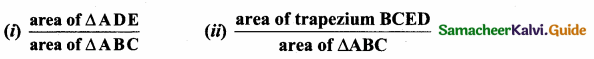Solution:
(i) Given, DE || BC and ADBD = 35
Let AD = 3k and BD = 5k; AB = 3k + 5k = 8k
∠A = ∠A (common angle)
Since DE || BC
∴ ∆ ABC ~ ∆ ADE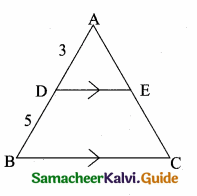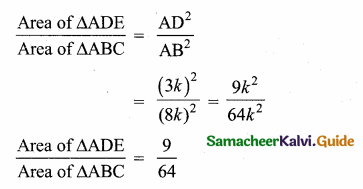(ii) Let Area of ∆ ADE be 9k and Area of ∆ ABC be 64 k
Area of ∆ BCED = Area of ∆ ABC – Area of ∆ ADE
= 64 k – 9 k
= 55 k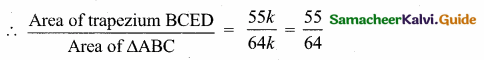Question 4.
A boy is designing a diamond shaped kite, as shown in the figure where AE = 16 cm, EC = 81 cm. He wants to use a straight cross bar BD. How long should it be?
Solution:
Let in AB be “x”, BE be “z” and BC be “y”
In the right ∆ AEB,
AB2 = AE2 + BE2
x2 = 162 + Z2 …….(1)
In the right ∆ BEC,
BC2 = EC2 + BE2
y2 = 812 + z2 ……(2)In the right ∆ ACD,
972 = x2 + y2 ….(3)
Add (1) and (2) ⇒ x2 + y2 = 162 + z2 + 812+ z2
x2 + y2 = 2z2 + 162 + 812
972 = 2z2 + 162 + 812 (from 3)
9409 = 2z2 + 256 + 6561
= 2z2 + 6817
2z2 = 9409 – 6817 = 2592
z2 = 25922 = 1296
z = 1296−−−−√ = 36
∴ Length of cross bar BD = 2 × BE = 2 × 36 = 72 cm

Question 5.
Find the unknown values in each of the following figures. All lengths are given in centimetres (All measures are not in scale)

Solution:
(i) In ∆ ABC and ∆ ADE,
∠A = ∠A (common angle)
∠ABC = ∠ADE (corresponding angle) [BC || DE]
∆ ABC ~ ∆ ADE (By AAA similarity)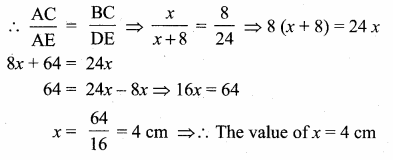In ∆ EAG and ∆ ECF,
∠E || ∠E (common angle)
∠ECF = ∠EAG (corresponding angle)
Given CF || AG
∆ EAG ~ ∆ ECF
ECEA = CFAG ⇒ 8x+8 = 6y ⇒ 812 = 6y (x = 4)
8y = 6 × 12
y = 12×68 = 9 cm
∴ The value of x = 4 cm and y = 9 cm.

(ii) In ∆ HBC and ∆ HFG,
∠H = ∠H (common angle)
∠HFG = ∠HBC (corresponding angle)
Given FG || BC
HFHB = FGBC ⇒ 410 = x9 ⇒ 10x = 36
x = 3.6 cm
In ∆ FBD and ∆ FHD,
∠BFD = ∠HFG (vertically opposite angle)
∠FBD = ∠FHG (Alternate angles)
By AA similarity
∆ FBD ~ ∆ FHG
FGFD = FHFB ⇒ x3+y = 46
4 (3 + y) = 3.6 × 6
3 + y = 3.6×64 = 5.4 ⇒ y = 5.4 – 3 = 2.4 cm
In ∆ AEG and ∆ ABC,
∠A = ∠A (common angle)
∠AEG = ∠ABC (corresponding angles)
Given EG || BC
AEAB = EGBC
zz+5 = x+y9 ⇒ zz+5 = 3.6+2.49
zz+5 = 69 ⇒ zz+5 = 23
3z = 2z + 10 ⇒ 3z – 2z = 10 ⇒ z = 10
∴ The values of x = 3.6 cm, y = 2.4 cm and z = 10 cm.

Question 6.
The internal bisector of ∠A of AABC meets BC at D and the external bisector of ∠A meets BC produced at E. Prove that BDBE = CDCE
Solution:
Given: In ∆ ABC, AD is the internal bisector of ∠A meets BC at D. AE is the external bisector of ∠A meets BC produced to E.
To Proof: BDBE = CDCE

Proof: In ∆ ABC, AD is the internal bisector of ∠A.
By ABT theorem we have,
∴ BECD = ABAC ……….(1)
In ∆ABC, AE is the external bisector of ∠A.
By ABT theorem we have,
∴ BECE = ABAC ……….(2)
From (1) and (2) we get
BDCD = BECE ⇒ ∴ BDBE = CDCE

Question 7.
In a quadrilateral ABCD, the bisectors of ∠B and ∠D intersect on AC at E. Prove that ABBC = ADDC
Solution:
Given: ABCD is a quadrilateral. BE is the bisector of ∠B intersecting AC at E, DE is the bisector of ∠D intersecting AC at E.
Proof: In ∆ABC, BE is the internal bisector of ∠D.
By Angle bisector theorem we have,
AEEC = ABBC ……..(1)

Question 9.
D is the midpoint of the side BC of AABC. If P and Q are points on AB and on AC such that DP bisects ∠BDA and DQ bisects ∠ADC, then prove that PQ || BC.
Solution:
In ∆ABD,DP is the angle bisector of ∠BDA.
∴ APPB = ADBD (angle bisector theorem) ……..(1)
∴ AQQC = ADDC (angle bisector theorem) ……….(2)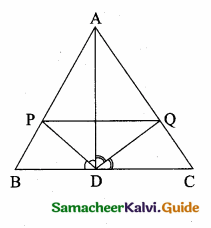But, BD = DC (D is the midpoint of BC)
Now (2) ⇒ ∴ AQQC = ADBD
From (1) and (3) We get,
∴ APPB = AQQC
Thus PQ || BC (converse of thales theorem)

Also given ∆ DEA ~ ∆ CEB
DECE = EAEB = DACB
DECE = EAEB …………(2)
From (1) and (2) we get
BEEA = EAEB ⇒ EB2 = EA2
∴ EB = EA
Substitute in (2) we get
EAEA = DACB
1 = DACB ⇒ ∴ AD = DC Hence it is proved

#### VISITORS COUNTUsers Today : 244Total Users : 380102Views Today : 667Total views : 1325867